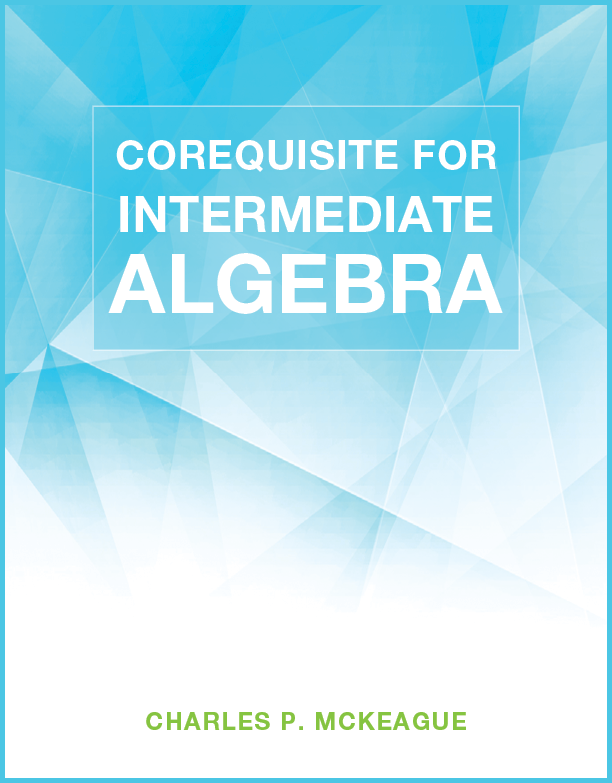## Corequisite for Intermediate Algebra

### Author: Charles P. McKeagueThis book contains everything you need to make a successful start in Intermediate Algebra

Here are the lessons:

Lesson 1: Fundamental Definitions and Notation
Lesson 2: Properties of Real Numbers
Lesson 3: Arithmetic with Real Numbers
Lesson 4: Exponents and Scientific Notation
Lesson 5: Polynomials, Sums, Differences, and Products
Lesson 6: Factoring
Lesson 7: More Factoring
Lesson 8: Linear and Quadratic Equations
Lesson 9: Formulas
Lesson 10: Linear Inequalities in One Variable
Lesson 11: Paired Data and the Rectangular Coordinate System
Lesson 12: The Slope of a Line
Lesson 13: Finding the Equation of a Line
Lesson 14: Systems of Equations in Two Variables
Lesson 15: Definitions and Common Roots